# Create and use summary tables

A summary table, also called a pivot table, uses groups and statistics to transform your raw data into a more accessible format. You can use a summary table to calculate counts and statistics—including sum, average, minimum, maximum, median, and percentile—for unique categories in your data using the number fields in your dataset. The same number field can be used multiple times to calculate all of the available statistics. Subgrouping and sorting features allow you to explore your data further to gain insights that aren't available in the raw table format.

## Example

A marketing researcher is planning a campaign to promote colleges with a high return on investment. As part of the research, she creates a summary table showing the average cost of colleges for each region in the United States to see how the costs compare across regions.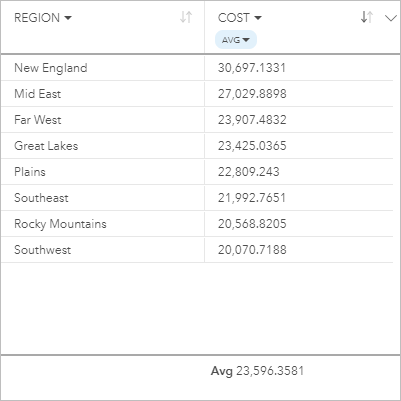## Create a summary table

Complete the following steps to create a summary table:

1. Select the fields to display in the summary table.

There is no limit to the number of fields that can be used in a summary table. String, number, and rate/ratio fields are supported in summary tables. If you use a location field, the display field is used in the summary table. Date/time fields cannot be used in summary tables.

##### Tip:

You can search for fields using the search bar in the data pane.

2. Create the summary table using the following steps:
1. Drag the selected fields to a new card.
2. Hover over the Table drop zone.
3. Drop the selected fields on Summary Table.
##### Tip:

You can also create summary tables using the Table button above the data pane or the Visualization type button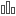on an existing card. Only compatible visualizations (including maps, charts, or tables) are listed in the Visualization type menu.

## Usage notes

You can choose a summary statistic for each number or rate/ratio field being summarized. The available statistics are sum, minimum, maximum, average, median, and percentile. You can customize the percentile statistic using the text box on the menu. For example, to view the 90th percentile, type 90 in the text box.

##### Note:

The median and percentile statistics are not available for certain remote feature layers. If your remote feature layer does not support median or percentile, you can copy the layer to your workbook.

The chosen statistic is calculated for each row on the summary table, as well as the entire dataset. The dataset statistic is displayed as a footer in the summary table.

The dataset statistic is calculated using the raw dataset, rather than the values from the summary table. This distinction is most important for the average statistic. For example, if you have a dataset with 10,000 features and you create a summary table that has 5 rows, the dataset average is calculated using all 10,000 features, rather than averaging the 5 rows in the table.

Use the Visualization type buttonto switch directly between a summary table and other visualizations, such as a bar chart or reference table. The visualization type options depend on the fields used to create the summary table.

Use the Flip card button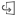to view the back of the card. The Card info tab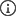provides information about the data on the card and the Export data tab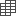allows users to export the data from the card.

When you create a summary table, a result dataset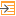with the string and number fields used to create the table is added to the data pane. You can use the result dataset to find answers with nonspatial analysis using the Action button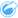.

##### Note:

If you need to add a calculated field to a summary table, the field must be calculated in the original dataset used to create the summary table, not the result dataset. The field will appear in the result dataset after it is added to the summary table.